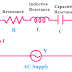In this article, we are going to know about the very important terms in electrical circuit theory i.e., Resistance and Reactance. Difference between Resistance and Reactance. We will try to understand the theoretical concept only and will know their definition, units, and the relation between them.
Except for Resistance, the rest all are related to AC circuits only. Some of them are properties of a conductor or object, and others are imaginary parts only.

## What is Resistance?

In simple words, resistance is a property of an object by virtue of which it opposes the flow of electrical current, which is called resistance.

When we applied a potential difference across an object, an electric current flowing through it if it is a conductor otherwise no current will flow if it is an insulator. Although, there is no ideal insulator that can completely oppose the flow of electrical current and no ideal conductor which can conduct electrical current with zero resistance.

Anyway, the resistance property of an object mainly depends upon the material it is made of. The shape and size of the object also an important factor for the resistance property. Another important factor, the resistance property depends on is temperature. Yes, an object can show different resistance properties at different temperatures. Remember that, resistance property does not affect by type of electricity means it shows the same effect for both AC and DC.

## Symbol and Unit of Resistance

The SI unit of the electrical resistance is Ohm and it is denoted by the symbol 'Ω'

## Resistance effect in Electrical or Electronic Circuit

1. Due to the resistance effect, electrical current experiences opposition to flow.

2. Due to the resistance effect, an electrical voltage drop occurs in electrical and electronics circuits.

3. Due to the resistance effect, electrical power dissipation or power loss happens.

4. Due to the resistance effect, electrical energy converted into heat energy.

## Application of Resistance

1. Electric Heater is the best example or application of resistance effect. Here the resistance property of the heater coil is used to convert the electrical energy into heat energy.

2. Resistor is a very well known and widely used passive component. We can see its application everywhere in electrical and electronic circuits.

## What is Reactance?

The opposition property of an element or component to the flow of electrical current due to its inductive or capacitive property is called Reactance or Electrical Reactance.

The opposition to electric current due to inductance or capacitance effect in an electrical or electronic circuit is also called Reactance. So the greater reactance effect causes the smaller current to flow for the same applied voltage.

## Symbol and Unit of Reactance

The unit of electrical reactance is Ohm but it is denoted by the symbol of 'X'

## Electrical Reactance Example

The passive elements or components such as inductors and capacitors can oppose the electric current due to their inductive and capacitive effect. This is an example of the reactance effect caused by electronic components.

Another example of the reactance effect caused by inductance and capacitance effect is the transmission line and magnetic coil.
We can see the capacitance effect in transmission lines and the inductance effect in magnetic circuits.

## Effect of Reactance in Electrical or Electronic Circuit

1. Generally, the reactance effect occurs in the AC circuits because the inductance and capacitance effect occurs in AC circuits only. So, due to the reactance effect, alternating current experiences the opposition to flow.

2. Due to the reactance effect, the power factor of the AC circuit will vary, for example, if there inductance effect is more than the capacitance effect in a circuit then the nature of the reactance will be inductive which causes a low power factor.

3. Due to the reactance effect, unwanted noise, impulse voltage occur in electrical and electronic circuits.

## Applications of Reactance

1. The inductive reactance effect is used in AC circuits to give protection against surge voltage. Using of Reactors in electrical power transmission and distribution network are the example.

2. Both inductive and Capacitive reactance effect is used in electronic communication or telecommunication circuits.

3. The reactance of a circuit consisting of both inductive and capacitive elements, is used to compute or analyze the amplitude, nature of power factor, changes in the phase of that circuit.

## Resistance VS Reactance

1. Resistance is the property of an object to oppose the flow of electrical current due to its nature and the shape of the material is made of, whereas Reactance is the property of an element or component to oppose the flow of electric current due to its inductance or capacitance effect.

2. Resistance can oppose the flow of current only, it cannot make any changes in the phase whereas the reactance can make changes in the phase for which current flowing through the element shifted relative to the voltage applied across the element.

3. Another very important difference between Resistance and Reactance is, resistance causes losses of electrical energy in the form of heat energy whereas Reactance does not any losses, even it can store energy in form of a magnetic field or electric charge and can again return to the circuit.

4. Reactance can be negative, so we can reduce the reactance effect in a circuit by creating its opposite or negative reactance but in the case of Resistance, this is not possible.

5. Resistance work in both AC and DC circuits whereas Reactance work in AC circuit only because the inductance and capacitance effect happens in AC circuit only.

Resistance Reactance Difference, Effects, Example, ApplicationReviewed by manoranjan das on 2/02/2021 Rating: 5